Next: The isothermal atmosphere Up: Classical thermodynamics Previous: Isothermal and adiabatic expansion

## Hydrostatic equilibrium of the atmosphere

The gas which we are most familiar with in everyday life is, of course, the Earth's atmosphere. In fact, we can use the isothermal and adiabatic gas laws to explain most of the observable features of the atmosphere.

Let us, first of all, consider the hydrostatic equilibrium of the atmosphere. Consider a thin vertical slice of the atmosphere of cross-sectional areawhich starts at heightabove ground level and extends to height. The upwards force exerted on this slice from the gas below is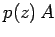, where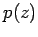is the pressure at height. Likewise, the downward force exerted by the gas above the slice is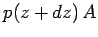. The net upward force is clearly. In equilibrium, this upward force must be balanced by the downward force due to the weight of the slice: this is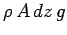, whereis the density of the gas, and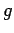is the acceleration due to gravity. In follows that the force balance condition can be written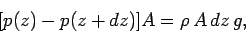(322)

which reduces to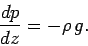(323)

This is called the equation of hydrostatic equilibrium for the atmosphere.

We can write the density of a gas in the following form,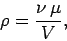(324)

where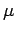is the molecular weight of the gas, and is equal to the mass of one mole of gas particles. For instance, the molecular weight of Nitrogen gas is 28 grams. The above formula for the density of a gas combined with the ideal gas law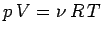yields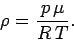(325)

It follows that the equation of hydrostatic equilibrium can be rewritten(326)Next: The isothermal atmosphere Up: Classical thermodynamics Previous: Isothermal and adiabatic expansion
Richard Fitzpatrick 2006-02-02